# MCQ in Electrical Circuit Part 16 | ECE Board Exam

(Last Updated On: November 24, 2020)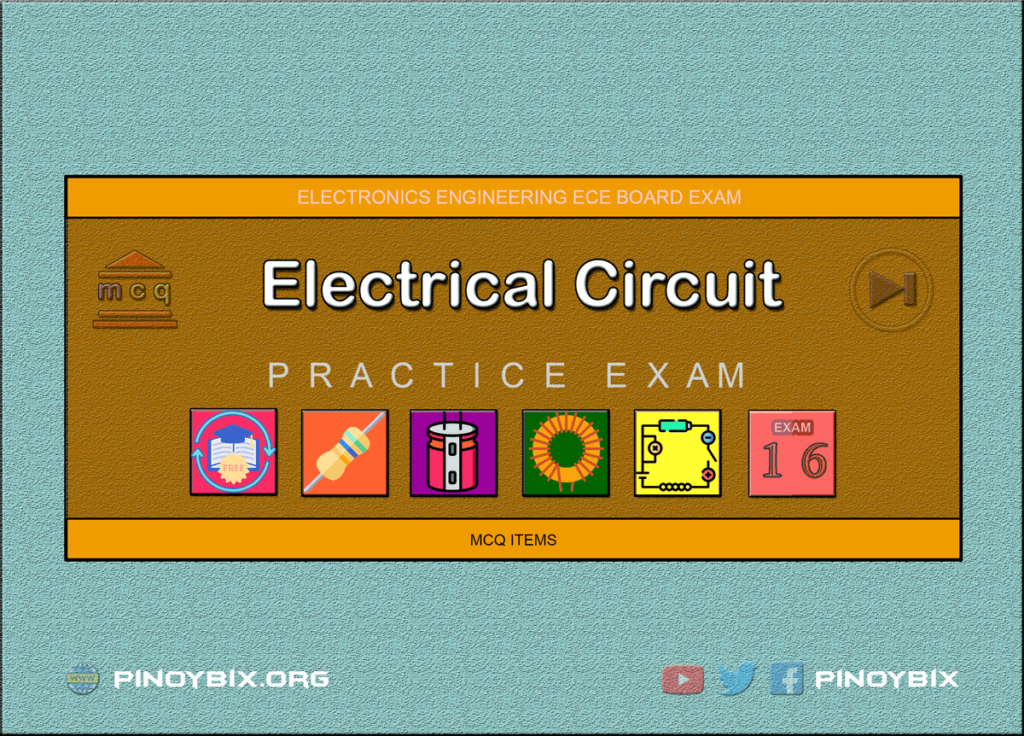This is the Multiples Choice Questions Part 16 of the Series in Electrical Circuit as one of the Electronics Engineering topic. In Preparation for the ECE Board Exam make sure to expose yourself and familiarize in each and every questions compiled here taken from various sources including but not limited to past Board Exam Questions in Electronics Engineering field, Electronics Books, Journals and other Electronics References.

#### MCQ Topic Outline included in ECE Board Exam Syllabi

• MCQ in AC-DC circuits
• MCQ in Resistors
• MCQ in Inductors
• MCQ in Capacitors

#### Continue Practice Exam Test Questions Part 16 of the Series

Choose the letter of the best answer in each questions.

751. Which of the following statement is TRUE both for a series and parallel DC circuit?

A. powers are additive

B. voltages are additive

C. current additive

D. elements have individual currents

View Answer:

Answer: Option A

Solution:

752. A 100-W, 110-V and a 50-W lamp are connected in series across a 220-V dc source. If the resistances of the two lamps are assumed to remain constant, the voltage across the 100-W lamp is ________ volt.

A. 110

B. 73.3

C. 146.7

D. 220

View Answer:

Answer: Option A

Solution:

753. In the parallel circuit of Fig.16.1, the value of V0 is ________ volt.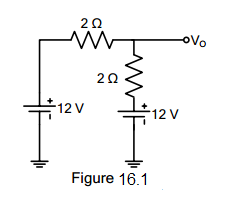electrical circuit figure 16.1

A. 12

B. 24

C. 0

D. -12

View Answer:

Answer: Option C

Solution:

754. In the series circuit of Fig 16.2, the value of V0 is ___________ volt.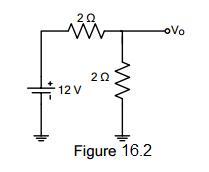electrical circuit figure 16.2

A. 12

B. -12

C. 0

D. 6

View Answer:

Answer: Option D

Solution:

755. In Fig 16.3, there is a drop of 20 V on each resistor. The potential of point A would be _________ volt.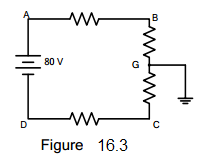electrical circuit figure 16.3

A. +80

B. -40

C. +40

D. -80

View Answer:

Answer: Option C

Solution:

756. From the voltmeter reading of Fig. 16.4, is it obvious that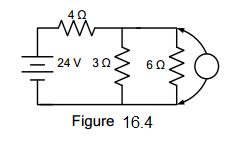electrical circuit figure 16.4

A. the 3 Ω resistor is short circuited

B. the 6 Ω resistor is short circuited

C. nothing is wrong with the circuit

D. the 3 Ω resistor is open-circuited

View Answer:

Answer: Option D

Solution:

757. With reference to Fig 16.5, which of the following statement is true?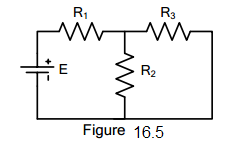electrical circuit figure 16.5

A. E and R1 form a series circuit

B. R1 is in series with R3

C. R1 is in series with R2

D. there is no series circuit

View Answer:

Answer: Option A

Solution:

758. Which of the following statements is correct concerning the Fig. 16.6?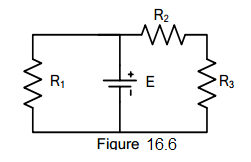electrical circuit figure 16.6

A. R2 and R3 form a series of path

B. E is in series with R1

C. R1 is in parallel in R3

D. R1, R2 and R3 form a series of circuit

View Answer:

Answer: Option B

Solution:

759. What is the equivalent resistance in ohms between points A and B of Fig. 16.7? All resistances are in ohms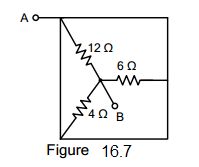electrical circuit figure 16.7

A. 12

B. 14.4

C. 22

D. 2

View Answer:

Answer: Option D

Solution:

760. What do you call a resistor that does not obey Ohm’s Law?

A. Potentiometer

B. Carbon-Film Resistor

C. Wire-Wound Type

D. Non-linear Resistor

View Answer:

Answer: Option D

Solution:

761. A 100 W, 110 V and 50 W, 110 V lamps are connected in series across a 220 V DC source. If the resistances of the two lamps are assumed to remain constant, the voltage across the 100 W lamp is _________ volt?

A. 110 V

B. 73.3 V

C. 146.7 V

D. 220 V

View Answer:

Answer: Option B

Solution:

762. A potential divider of resistance of 50 ohms is connected across a 100 V DC source. A load resistance of 10 ohms is connected across a tap in the potential divider and the negative terminal of the source. If a current of 4 A flows towards the load, what is the current supplied by the source?

A. 5.32 A

B. 5.05 A

C. 5.21 A

D. 5.48 A

View Answer:

Answer: Option B

Solution:

763. Two resistors A and B made of different materials have temperature coefficients of resistance at 20°C of 0.004 and 0.006 respectively. When connected across a voltage source at 20°C, they draw current equally. What percentage of the total current at 100°C does resistor A carry?

A. 47.14%

B. 52.86%

C. 61.34%

D. 38.66%

View Answer:

Answer: Option B

Solution:

764. A conductor has a resistance of 7 ohms at 0C. At 20°C, the resistance has become 7.5 ohms. Calculate the temperature coefficient of the conductor at 20°C.

A. 1/300 /°C

B. 1/400 /°C

C. 1/500 /°C

D. 1/600 /°C

View Answer:

Answer: Option A

Solution:

765. Which of the following is a non-linear element?

A. diode

B. heater coil

C. transistor

D. electric arc with unlike electrode

View Answer:

Answer: Option A

Solution:

766. EE Board Exam April 1992

An electric kettle was marked 500 W, 230 V found to take 15 minutes to bring 1 kilogram of water at 15°C to boiling point. Determine the heat efficiency of the kettle.

A. 79.1%

B. 75.3%

C. 72.4%

D. 74.8%

View Answer:

Answer: Option A

Solution:

767. REE Board Exam October 1997

A process equipment contains 100 gallons of water at 25°C. It is required to bring it to boiling in 10 minutes. The heat loss is estimated to be 5%. What is the kW rating of the heater?

A. 125 kW

B. 252 kW

C. 50.5 kW

D. 207 kW

View Answer:

Answer: Option D

Solution:

768. EE October 1989

A total of 0.8 kg of water at 20°C is placed in a 1-kW electric kettle. How long a time in minute is needed to raise the temperature of the water to 100°C?

A. 4.46 min

B. 5.32 min

C. 5.34 min

D. 4.56 min

View Answer:

Answer: Option A

Solution:

769. REE October 1998

How many calories does an electric heater of 100 watts generate per second?

A. 10

B. 1000

C. 23.88

D. 42.25

View Answer:

Answer: Option C

Solution:

770. REE Board Exam October 1997

The electric energy required to raise the temperature of water in a pool is 1000 kWh. If the heat losses are 25%, the heating energy required will be ___________.

A. 1111 kWh

B. 1266 kWh

C. 1750 kWh

D. 1333 kWh

View Answer:

Answer: Option D

Solution:

771. EE Board Exam April 1992

An electric heater carries 12 A at 110 V, is submerged in 22.5 lbs of water for 30 minutes. What will be the final temperature of the water if its initial temperature is 35°F?

A. 135.43°F

B. 125.42°F

C. 133.56°F

D. 128.33°F

View Answer:

Answer: Option A

Solution:

772. EE Board Exam October 1990

In an electric heater the inlet temperature is 15°C. Water is flowing at the rate of 300 grams per minute. The voltmeter measuring voltage across the heating element reads 120 volts and an ammeter measuring current taken reads 10 amperes. When steady state is finally reached, what is the final reading of the outlet thermometer?

A. 57.6°C

B. 68.4°C

C. 72.6°C

D. 42.6°C

View Answer:

Answer: Option C

Solution:

773. EE Board Exam October 1991

Four cubic meters of water is to be heated by means of four 1.5 kW, 230-V immersion heating elements. Assuming the efficiency of the heater as 90%, determine the time required in boiling the water if the initial temperature is 20°C and if all four elements are connected in parallel.

A. 71 hrs

B. 63 hrs

C. 69 hrs

D. 66 hrs

View Answer:

Answer: Option C

Solution:

774. EE Board Exam October 1991

Four cubic meters of water is to be heated by means of four 1.5 kW, 230-V immersion heating elements. Assuming the efficiency of the heater as 90%, determine the time required in boiling the water if the initial temperature is 20°C and if the elements are connected two in series in parallel with two in series.

A. 275.6 hrs

B. 295.3 hrs

C. 252.2 hrs

D. 264.4 hrs

View Answer:

Answer: Option A

Solution:

775. REE Board Exam September 2001

How many joules per second are then in 10 watts?

A. 10

B. 5

C. 20

D. 24.5

View Answer:

Answer: Option A

Solution:

776. REE Board Exam September 2001

10^13 ergs/sec is equal to how many kilowatts?

A. 1,000

B. 250

C. 100

D. 10

View Answer:

Answer: Option A

Solution:

777. REE Board Exam September 2000

What is 1 kW-hr in BTU?

A. 4,186

B. 3,413

C. 746

D. 1,000

View Answer:

Answer: Option B

Solution:

778. REE Board Exam October 1998

What is the work in ergs needed to raise a 10 g weight 100 m up?

A. 4.9 x 10^5

B. 9.8 x 10^7

C. 98 x 10^7

D. 1.96 x 10^7

View Answer:

Answer: Option B

Solution:

779. REE Board Exam October 1999

The quantity of heat required to raise the temperature of water by 1°C.

A. energy

B. specific heat

C. calorie

D. BTU

View Answer:

Answer: Option C

Solution:

780. REE Board Exam October 1999

When heat is transferred into any other form of energy or when other forms of energy are converted into heat, the total amount of energy is constant. This is known as

A. First law of thermodynamics

B. Boyle’s law

C. Specific heat

D. Isothermal expansion

View Answer:

Answer: Option A

Solution:

781. REE Board Exam September 2002

What is 10^12 ergs/sec in kW?

A. 100 kW

B. 1,000 kW

C. 10 kW

D. 10,000 kW

View Answer:

Answer: Option A

Solution:

782. REE Board Exam October 2000

An electric heater is used to heat up 600 grams of water. It takes 14 minutes to raise the temperature of water by 40°C. If the supply voltage is 220 volts,what is the power rating of the heater neglecting heat losses?

A. 180 W

B. 120 W

C. 200 W

D. 60 W

View Answer:

Answer: Option B

Solution:

783. REE Board Exam April 2001

A 100 liter of water is heated from 20°C to 40°C. How many kWHR of electricity is needed assuming no heat loss?

A. 4.2

B. 2.3

C. 5.6

D. 3.7

View Answer:

Answer: Option B

Solution:

784. REE Board Exam April 2002

Ten (10) kW is equal to __________ gram-cal/sec.

A. 156

B. 436

C. 2,388

D. 425

View Answer:

Answer: Option C

Solution:

785. ECE Board Exam November 1995

Two heaters A and B are in parallel across supply voltage V. Heater A produces 500 kcal in 20 minutes and B produces 1000 kcal in 10 minutes. The resistance of A is 10 ohms. What is the resistance of B, if the same heaters are connected in series voltage V?

A. 4.5 ohms

B. 2.5 ohms

C. 4.5 ohms

D. 0.14 ohm

View Answer:

Answer: Option B

Solution:

786. In the SI system of units, the unit of force is

A. kg-wt

B. Newton

C. Joule

D. N-m

View Answer:

Answer: Option B

Solution:

787. The basic unit of electric charge is

A. ampere-hour

B. watt-hour

C. coulomb

D. farad

View Answer:

Answer: Option C

Solution:

788. The SI unit of energy is

A. Joule

B. kWh

C. kcal

D. m-kg

View Answer:

Answer: Option A

Solution:

789. Two heating elements, each of 230-V, 3.5 kW rating are first joined in parallel and then in series to heat same amount of water through the same range of temperature. The ratio of the time taken in the two cases would be

A. 1:2

B. 2:1

C. 1:4

D. 4:1

View Answer:

Answer: Option C

Solution:

790. If a 220 V heater is used on 110 V supply, heat produced by it will be _________ as much

A. one-half

B. twice

C. one-fourth

D. four times

View Answer:

Answer: Option C

Solution:

791. For a given line voltage, four heating coils will produce maximum heat when connected

A. all in parallel

B. all in series

C. with two parallel pairs in series

D. one pair in parallel with the other two in series

View Answer:

Answer: Option A

Solution:

792. The electric energy required to raise the temperature of a given amount of water is 1000 kWh. If heat losses are 25%, the total heating energy required is ____________ kWh.

A. 1500

B. 1250

C. 1333

D. 1000

View Answer:

Answer: Option C

Solution:

793. One kWh of energy equals nearly

A. 1000 W

B. 860 kcal

C. 4186 J

D. 735.5 W

View Answer:

Answer: Option B

Solution:

794. A force of 10,000 N accelerates a body to velocity 0.1 km/s. The power developed is __________ kW.

A. 1,000,000

B. 36,000

C. 3600

D. 1000

View Answer:

Answer: Option D

Solution:

795. A 100 W light bulb burns on an average of 10 hours a day for one week. The weekly consumption of energy will be ______________ unit/s.

A. 7

B. 70

C. 0.7

D. 0.07

View Answer:

Answer: Option A

Solution:

796. Two heaters, rated at 1000 W, 250 volts each, are connected in series across a 250 volt, 50 Hz A.C. mains. The total power drawn from the supply would be __________ watt.

A. 1000

B. 500

C. 250

D. 2000

View Answer:

Answer: Option B

Solution:

797. One watt is equal to ___________.

A. 4.19 cal/sec

B. 778 BTU/sec

C.10^7 ergs/sec

D. 10^-7 ergs/sec

View Answer:

Answer: Option C

Solution:

798. The current in an electric lamp is 5 amperes. What quantity of electricity flows toward the filament in 6 minutes?

A. 30 C

B. 3600 C

C. 72 C

D. 1800 C

View Answer:

Answer: Option D

Solution:

799. An electric heater is rated at 120 volts, 1000 watts and is used to boil water. Calculate the time in minutes to raise the temperature of 1 liter of water from 15°C to boiling. The heater has an over-all efficiency of 92%.

A. 6.4 minutes

B. 5.4 minutes

C. 4.4 minutes

D. 3.4 minutes

View Answer:

Answer: Option A

Solution:

800. For a given line voltage, four heating coils will produce maximum heat when connected

A. all in parallel

B. all in series

C. with two parallel pairs in series

D. one pair in parallel with the other two in series

View Answer:

Answer: Option B

Solution:

#### Questions and Answers in Electrical Circuit

Following is the list of practice exam test questions in this brand new series:

MCQ in Electrical Circuit
PART 1: MCQ from Number 1 – 50                        Answer key: PART 1
PART 2: MCQ from Number 51 – 100                   Answer key: PART 2
PART 3: MCQ from Number 101 – 150                 Answer key: PART 3
PART 4: MCQ from Number 151 – 200                 Answer key: PART 4
PART 5: MCQ from Number 201 – 250                 Answer key: PART 5
PART 6: MCQ from Number 251 – 300                 Answer key: PART 6
PART 7: MCQ from Number 301 – 350                 Answer key: included
PART 8: MCQ from Number 351 – 400                 Answer key: included
PART 9: MCQ from Number 401 – 450                 Answer key: included
PART 10: MCQ from Number 451 – 500                 Answer key: included

### Complete List of MCQ in Electronics Engineering per topic

Please do Subscribe on YouTube!

P inoyBIX educates thousands of reviewers and students a day in preparation for their board examinations. Also provides professionals with materials for their lectures and practice exams. Help me go forward with the same spirit.

“Will you subscribe today via YOUTUBE?”

Subscribe